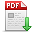# 魏超峰,李琼林,蒋建东,等.计及谐波因素的配电网损耗量化计算与建模仿真[J].郑州大学学报(工学版),2018,39(01):53. 　Quantification Calculation and Modeling Simulation of Distribution Network Losses Considering Harmonic Factor[J].Journal of Zhengzhou University (Engineering Science),2018,39(01):53. 点击复制 计及谐波因素的配电网损耗量化计算与建模仿真() 分享到： var jiathis_config = { data_track_clickback: true };

39

2018年01期

53

2018-01-20

## 文章信息/Info

Title:
Quantification Calculation and Modeling Simulation of Distribution Network Losses Considering Harmonic Factor

A

Abstract:
Accurate line loss calculation is the basis of realizing economic operation of power grid. The harmonic pollution in distribution network is becoming increasingly serious. Because of harmonic factors, the distribution network operation state and equipment parameters have changed greatly, and the additional losses arising therefrom have not been neglected. Based on the analysis of the traditional calculation method of line loss, this paper systematically analyzes the mechanism of harmonic effect on distribution transformer and power line. By introducing the transmission line AC resistance coefficient and transformer harmonic loss factors, a loss calculation model considering harmonic is put forward. Then, combined with the typical distribution transformer and transmission line, the harmonic loss was calculated quantitatively. Finally, the validity and accuracy of the model are verified by modeling and simulation.